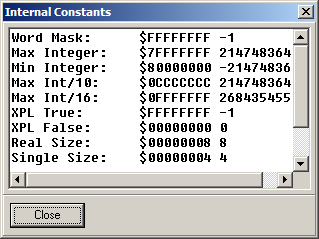﻿ Internal Constants
 Internal Constants
 The Internal Constants dialog box is accessed by selecting the Tools -> Internal Constants option from the menu bar.The Internal Constants are used by the language to control the various aspects of the program. They are used to set the size of integers, format the displaying of numbers, and check for overflow conditions. Since this version of EXPL can be configured for either 16- or 32-bit operation, the values reflect how the language is configured. Here is a complete description of each item:   Word Mask: This is a bit mask that can be "anded" with a number to reduce it to the size of an XPL integer. Max Integer: This is the maximum positive value of a signed XPL integer. Min Integer: This is the minimum negative value of a signed XPL integer. Max Int/10: This is the maximum integer divided by 10. It is used to anticipate the overflow of numbers as they are input. Max Int/16: This is the maximum unsigned integer divided by 16. It is used to anticipate the overflow of hex numbers as they are input. XPL True: This is the numerical value of the XPL "true" constant. XPL False: This is the numerical value of the XPL "false" constant. Real Size: This is the size in bytes of the XPL real number type. Single Size: This is the size in bytes of the XPL short real number type. Int Bytes: This is the size of the XPL integer in bytes. Int Nibbles: This is the size of the XPL integer in nibbles.